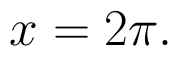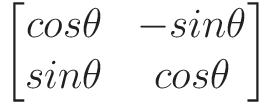Select Page

# CBSE Maths 12 Science Vector Algebra MCQ Solutions in English

CBSE Maths 12 Science Vector Algebra MCQ Solutions in English to enable students to get Solutions in a narrative video format for the specific question.

Expert Teacher provides CBSE Maths 12 Science Vector Algebra MCQ Solutions through Video Solutions in English language. This video solution will be useful for students to understand how to write an answer in exam in order to score more marks. This teacher uses a narrative style for a question from Vector Algebra not only to explain the proper method of answering question, but deriving right answer too.

Please find the question below and view the Solution in a narrative video format.

Question:

Solution Video in English:

You can select video Solutions from other languages also. Please check Solutions in ( Hindi )

## Similar Questions from CBSE, 12th Science, Maths, Vector Algebra

Question 1 : Find |x|, if for a unit vector a,(x - a).(x + a) = 12. (View Answer Video)

Question 2 :  Find the scalar quantities from the following:
(i) 10 kg          (ii) 2 m north-west          (iii)(iv) 40 W         (v)(vi)(View Answer Video)

Question 3 : Find the sum of the vectorsand(View Answer Video)

Question 4 : Find the scalar quantities :
(i)     Work                     (ii)      Force

Question 5 : Find the scalar quantities from the following:
(i) Time period          (ii) Distance          (iii) Force

### Application of Integrals

Question 1 : Using integration, find the area of the triangle ABC, where A is (2,3), B is (4,7) and C is (6,2). (View Answer Video)

Question 2 : Find the area enclosed between the parabolaand the line y = mx. (View Answer Video)

Question 3 : Using the method of integration, find the area of the region bounded by the lines 3x - y - 3 = 0, 2x + y - 12 = 0 and x -2y - 1 = 0. (View Answer Video)

Question 4 : Find the area bounded by the curve y = sin x between x = 0 and(View Answer Video)

Question 5 : Find the area bounded by curvesand(View Answer Video)

### Integrals

Question 1 : Find. (View Answer Video)

Question 2 :(View Answer Video)

Question 3 : Evaluate :(View Answer Video)

Question 4 : Evaluate :. (View Answer Video)

Question 5 : Find :(View Answer Video)

### Determinant

Question 1 : Evaluate  the determinants in :. (View Answer Video)

Question 4 : Evaluate  the determinants in :.(View Answer Video)
Question 5 : Find the adjoint  of the matrix. (View Answer Video)